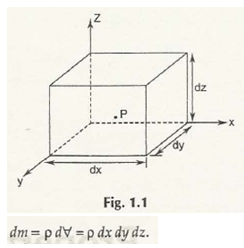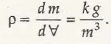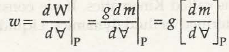The properties of fluids determine the nature of fluid under specific conditions like temperature and pressure. Some of the general properties of fluids include:

• Density or Mass Density:

The density specifies the quantity of matter presents in unit volume of substance. It is denoted by (rho) and its S.I. unit is kg/ m3. In fluid mechanics, density can be expressed as shown in Fig. 1.1.Let us consider dm be the mass of a fluid element and its volume is dV at point P, then we can write the equation as-Or,

= dm/ dV

Note:

Density of water is 1000 kg/m3

Density of mercury is 13593 kg/m3

Density of air is 1.23 kg/m3

• Specific Weight or Weight Density:

Specific weight of a fluid element is defined as the ratio of weight per unit volume. It can also be termed as weight density. Specific weight is denoted by  (omega) and its unit is N/m3.

Mathematically, it can be expressed as-

Let us consider dW be the weight of fluid element and dV be its volumeHence,

= g .

Or,

=  . g

Note:

Specific weight of water is 9.81 x 103N/m3

Specific weight of mercury is 133.7 x 103N/m3

• Specific Gravity or Relative Density:

Specific Gravity is also termed as Relative Density is the ratio of density of the given substance to the density of standard substance or any reference substance. It is a dimensionless quantity and has no unit.

The reference substance is usually water at temperature 40C for all liquids and air at temperature 210C. For both these reference substances, pressure is taken as 1 atm or 101.325 kPa. It is denoted by S and mathematically, it can be written as-

S = liquid/ water

To calculate the gravity of liquid,

liquid = S x water

or,

liquid = S x 1000 kg/m3

S can also be expressed in the ratio of mass of one substance to the mass of any reference substance at same volume.

Mathematically,

S = liquid/ water

To calculate the gravity of liquid,

liquid = S x water

Or,

liquid = S x 9.81 x 103 N/m3

• Viscosity:

Viscosity determines the resistance to deformation of the substance with respect to shear stress or tensile stress. For different liquid, the value of viscosity differs at same shear stress.

Let us consider the velocity of fluid flow be u and shear stress be –

Viscosity of fluid can be given by,

To remove the sign of proportionality,

=

Where,

= Co-efficient of viscosity

Unit:

The S.I. unit of viscosity is N.s/m2

In some cases, it is also written as kg/m.s

Note:

The fluid which has high viscosity can be easily determined by referring to its thickness. It means water has low viscosity as compare to honey. In other words, high viscous fluids require more energy to make it flow as compare to low viscous fluids.

Links of Next Mechanical Engineering Topics:-### Customer Reviews

My Homework Help
Rated 5.0 out of 5 based on 510 customer reviews at